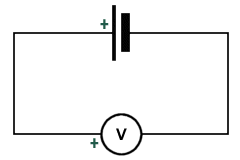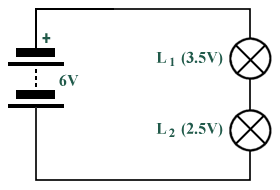Basic Electrical Theory

Overview

All matter is comprised of molecules. The molecule is the smallest quantity of a substance that can exist and still display all the physical and chemical properties of that substance. Molecules are made up of smaller particles called atoms of which there are over a hundred different types. All molecules consist of various combinations of atoms. For example, two atoms of hydrogen and one of oxygen form one molecule of water, and sulphuric acid is made up of two atoms of hydrogen, one of sulphur and four of oxygen. Atoms consist of a relatively heavy, positively charged core or nucleus, around which a number of much lighter negatively charged electrons move in one or more orbits. One type of atom differs from another in the number of protons (positively charged particles) and neutrons (particles that do not have either a positive or negative charge) which make up the nucleus, and in the number of negatively charged electrons which continually orbit the nucleus. The orbiting electrons are arranged in one or more orbits, depending on how many electrons the atom has. The more electrons there are, the greater the number of orbits. It is sometimes possible for an electron to become detatched from an atom, especially if it is the only electron in the outermost orbit.The structure of a copper atom

The ease with which electrons can be detached from their parent atoms varies from one substance to another. In some substances there is continual random movement of electrons from one atom to another, and the application of a voltage across a piece of wire made of such a substance (for example from a battery) will cause some of these free electrons to flow along the wire in one direction, producing an electric current. The electrons in the outermost orbit (or shell) of an atom are called valence electrons. If the outer shell has a full complement of electrons, it is very difficult to knock one of these valence electrons out of its orbit, and they are said to be tightly bound to the atom. Materials with such a structure do not conduct electricity easily (i.e. they are insulators). If there are only one or two valence electrons, however, the bond between the electron and the atom is much more easily broken, and there will be far more free electrons moving around within the material, making it a conductor.

Current, voltage and resistance

In metals such as copper, the valence electrons are held only loosely by their atoms and can move from one atom to another. The motion of these free electrons within the material is usually random, but if a force acts on the free electrons in a metal wire such that they all flow along the wire in the same direction, an electric current is produced. When this happens, the wire may get warm, and the needle of a magnetic compass brought into close proximity to the wire can be deflected because a magnetic field is created in the space surrounding the wire. A constant flow of electrons in one direction is called a direct current (abbreviated to dc).Electrons moving randomly (left) and electrons acted on by a force (right)

Current can be likened to the flow of water through a pipe, and is measured in amperes (A) by connecting an ammeter so that the current flows through it. The current is said to have a value of one ampere (1A) when approximately 6,000,000,000,000,000,000 (6 × 1018) electrons pass any point in a wire in one second. The currents used in the circuits that are found in most electronic devices tend to be very small. For this reason you will often come across current values expressed in smaller units such as milliamperes and microamperes. One milliampere (1 mA) is one thousandth (1 × 10-3) of an ampere. One microampere (1 µA) is one millionth (1 × 10-6) of an ampere. By convention, the direction of current flow is said to be from positive to negative (hence the term conventional current). Materials that conduct electricity are called conductors, and include all metals. Materials that do not conduct electricity are called insulators.

A battery can provide the force required to cause a current to flow in a wire. The battery produces an electromotive force (EMF) due to the chemical action that occurs inside it. Electromotive force is measured in volts (V) by connecting a voltmeter across the battery. Batteries produce direct current, and the EMF acts along a wire at almost the speed of light (3 × 108 metres per second) and causes the free electrons to drift in one direction around an electrical ciruit. The electrons themselves actually drift quite slowly, often travelling at less than one millimetre per second.EMF is measured across a battery or generator using a voltmeter

In the diagram below, lamps L1 and L2 are connected in series with a 6V battery. L1 is designed to be lit by a 3.5V supply, and L2 by a 2.5V supply.Two lamps connected in series with a 6V battery

The electro-motive force (emf) of the battery divides between the lamps so that each gets the correct supply. A voltmeter connected across L1 would read 3.5V and another voltmeter across L2 would read 2.5V. The portion of the e.m.f. appearing across each lamp is called the potential difference (pd) across the lamp. If E is the emf of a battery, and V1 and V2 are the potential differences across two appliances (e.g. lamps) in series with it, then:

E = V1 + V2

Occasionally, you may need to know the potential at a specific point in a circuit. In this situation, you chose a point in a circuit and say that it has zero potential (0V). The potentials at all other points are then stated with reference to it. In other words, the potential at any point is the potential difference between that point and the reference point. In electronic circuits, a point of zero potential is called ground (or common or earth). Electro-motive forces and potential differences are generally referred to as voltages.

Electrons move more easily through some materials than others when an EMF is applied to them. Opposition to current flow is called resistance. A conductor such as copper has a low resistance, and short thick wires have less resistance than long thin ones. Conductors designed to have a specific resistance are called resistors. An insulator has a very high resistance, and is a poor conductor. Plastic materials such as polythene and PVC are good insulators, and allow only tiny currents to pass even at high voltages. Resistance is stated in ohms (abbreviated to Ω - the upper case Greek letter Omega). Values for resistance tend to be large. For this reason you will often come across values for resistance that are expressed in larger units such as kilohm and megohm. One kilohm (1 kΩ) is one thousand ohms (1 × 103 Ω). One megohm is one million ohms (1 × 106 Ω).